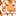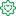# QlikView App Dev

Discussion Board for collaboration related to QlikView App Development.

Announcements
Skip the ticket, Chat with Qlik Support instead for instant assistance.
cancel
Showing results for
Did you mean:Contributor II

## Qlikview rounding up expression

I am using the following expression in Qlikview:   Dinar Detectives

``````=Num((if(SUM(EF)>= 100, num((((SUM(EF)-100)*0.0118)+12000), num(SUM(EF)*0,03,)))))
``````

I want to round the number. Like '€ #.##0'. How/where do I insert this into the expression? Can't seem to get it to work. Thanks in advance!

Labels (1)
• ### Other

3 RepliesContributor

I have exactly the same problem. Anyone got a suggestion Marriott Global SourceMVPFormer Employee

First of all, you have a large number of of non-matching brackets, so it is not quite clear what result you expect. Then the Num() calls inside your expression are not necessary. So I think you can simplify the existing expression to

Num(
if(
SUM(EF)>= 100,
(SUM(EF)-100)*0.0118+12000,
SUM(EF)*0.03
)
)

Now if you want to format this in Euro, you need to add parameters to the Num() function, e.g.

Num(
if(
SUM(EF)>= 100,
(SUM(EF)-100)*0.0118+12000,
SUM(EF)*0.03
),
'€ #.##0',
',',
'.'
)Community Browser